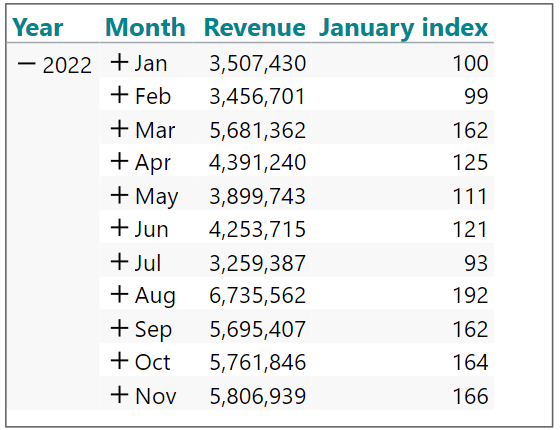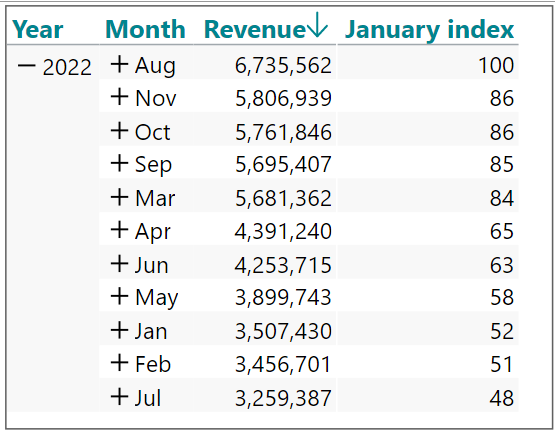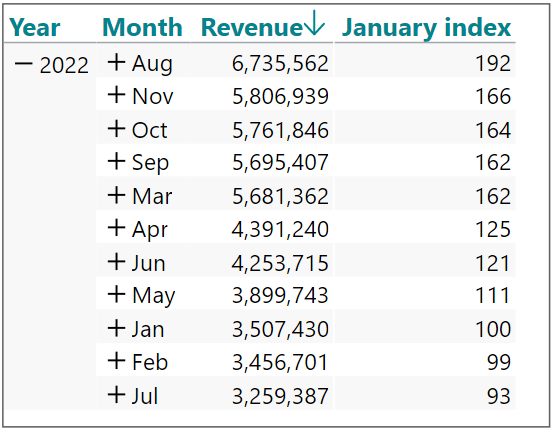# December 16th: Keep the original sequence for calculations - the magic of the (u) modifier

Sometimes the data sequence (sorting) can be important in a calculation.

Maybe even to the point where your calculation will break if someone (an end-user) sorts the data differently than the original sorting sequence.

Here is an example where this is the case.

In this table, we are calculating a January index which works when January is the first row.The syntax behind this index looks like this:

sum(d1, 0, m1) / sum(d1, d1, m1) * 100

Explanation:

The calculation looks at the current row (0) and divides that by the first row (d1), and multiplies by 100.

If the data is sorted by the end user, eg, in descending order based on the revenue, it will actually break the logic that supports the calculation:The January index has now turned into an August index.

To prevent this from happening, we can just add the (u) modifier to our original syntax.

sum(d1, 0, m1) / sum(d1, d1(u), m1) * 100

Now the sorted version of the crosstab will still calculate according to the original (unsorted) sequence of data:In conclusion, everywhere you refer to certain rows in any calculation, you should consider adding the (u) modifier.